PHYSICS Mass MASS MA. Fundamental of Mass MB. Kong Frequency and Kong Wavelength MC. Annihilation and Pair Production MD. Kong Equation ME. Kong Atom Model MF. Quantum of Atom MG. Perturbation of Photon MH. Periodic Table MI. Chemical Reaction MJ. Superconductor MK. Particles and Waves ML. Nuclear Physics ME. KONG ATOM MODEL 1. Magnetic Gauss Line 2. Kong Atom Model 3. Quantum Properties 5. Hydrogen Atom Model 6. X-Ray Spectrum 7. Comparison between Experiment and Calculation INTRODUCTION   In the chapter "Kong Frequency and Kong Wavelength", it describes that particles do not travel as wave, the wave model of atom requires to be refreshed. In the chapter "Annihilation and Pair Production", the structure of the particle is introduced. In this chapter, an alternative understanding on the structure of the atom is presented. Here, a new quantum quantity is introduced, which is the magnetic gauss line. The magnetic gauss line plays an important role to keep the electrons in the atom and to give the volumetric size of the atom. Electrons travel in the atom at certain velocity due to the balance of the magnetic and electric forces.   From the solutions of the Kong Equation and the brief atom model presented, here, we applied the solutions to the Kong atom model and to determine the quantum properties of the atom. The Time Dependent Kong Equation is used for the force balance between the atom and electrons. Based on the Kong atom model, proper methods are used to derive and develop the atom quantum properties such as the velocity of electron, the quantized radius, the magnetic gauss line, the magnetic dipole moment, the orbital angular momentum and the state energy.   The derivations are then verified with the hydrogen atom model and Moseley Plot.     OBJECTIVES   1)      To present an alternative atom model, the Kong Atom Model. 2)      To introduce another quantum quantity, the Kong Magnetic Gauss Line. 3)      To develop all the quantum mechanics for atoms. 4)      To verify all the quantum mechanics using hydrogen atom model and the Moseley Plot ME.1.0        MAGNETIC GAUSS LINE   ME.1.1        Exist of Magnetic Gauss Line   From the simple experiment of magnetic bar and iron powders, it shows that the iron powders is arranged nicely on certain path between the North and South Pole of the magnet bar.   For magnetic object such as magnet bar, the magnetic gauss line is the resultant of the superposition of all the magnetic dipole that properly arranged in the atomic level of the object. The path line shows a discrete energy that pulls the iron powders to arrange accordingly. The path line here is named as the magnetic gauss line. The magnetic gauss line which is shown by the iron powders possesses the shape of ellipsoidal toroid (donut).   Similarly, in atomic level, atoms shall also possess the discrete magnetic gauss line. Analogy to magnet bar, the magnetic gauss line appeared in the atomic level shall also possess the shape of ellipsoidal toroid, where the inner side of toroid is merged. The merged point of the toroid is the center of atom. The ellipsoidal toroid of magnetic gauss line of atom is proposed in figure ME.1.1.     ME.1.2        Gauss Law of Magnetism   In this chapter, we borrow the gauss’s law for magnetism and redefine it to the useful equation. The dot-product of Gauss’s law for magnetism is shown below,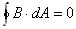… eq. ME.1.1 where   B = magnetic field dA = finite Gaussian surface   Eq. ME.1.1 is dimensionally imbalance, where at the right side, value zero is dimensionless. To make it balance, we need to insert a dimensioned factor on the left side. Two quantum quantities are introduced into eq. ME.1.1, which is the Plank’s constant and the charge. The dimensionally correct of Gauss’s law for magnetism should be as follow,… eq. ME.1.2 where   h = Plank’s constant q = charge that generates the magnetic field   Eq. ME.1.2 is used to develop the magnetic gauss line of the Kong atom model.     ME.1.3        Quantized Magnetic Gauss Line   Figure ME.1.1 shows an atom structure with magnetic gauss line for the first two principle orbit numbers. A spherical Gaussian surface is drawn along the magnetic gauss line of the atom. The Gaussian surface is then separated into three portions, which are the top, middle and bottom.   The top portion is the surface where the magnetic gauss line penetrates outwards from the Gaussian surface. The middle portion is the surface where the magnetic gauss line is parallel with the Gaussian surface. And the bottom portion is the surface where the magnetic gauss line penetrates inward from the Gaussian surface.Figure ME.1.1   From eq. ME.1.2, the overall magnetic gauss line that captured by the Gaussian surface is calculated below,… eq. ME.1.3   The atom model is symmetrical and the surface A1 and A3 are the same. The magnetic field across surface A1 is equal to the magnetic field across surface A3, but, in different direction, where,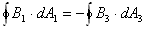… eq. ME.1.4   and the dot-product for surface A2 is,… eq. ME.1.5 where B2 = magnetic field of the magnetic gauss line that parallel to Gaussian surface.   Substitute eq. ME.1.4 and eq. ME.1.5 into eq. ME.1.3, it reduces to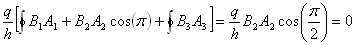… eq. ME.1.6   Upon the symmetrical shape of atom model, A1 = A3                                                                                                … eq. ME.1.7 and A = A1 + A2 + A3 = 2A1 + A2                                                            … eq. ME.1.8 where   A = 4pr2 = total Gaussian surface area, taken to be spherical surface.   The Gaussian surface that parallel to the magnetic gauss line is expressed as follow, A2 = k1A = 4k1pr2                                                                               … eq. ME.1.9 where k1 < 1, k1 = constant or partial of the spherical surface.   Substitute eq. ME.1.9 into eq. ME.1.6, we obtain… eq. ME.1.10   From eq. ME.1.10, we know that the properties of the radius (r), the magnetic field (B2), the charge (q) and the Plank’s constant (h) cannot be zero, therefore, we extract the non-zero terms to become,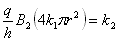… eq. ME.1.11 where k2 = constant or dimensionless function   Rearrange eq. ME.1.11, the magnetic gauss line has the value of… eq. ME.1.12 where   q = charge that generates the magnetic field K = k2 / k1 = the Kong function h = Plank’s constant r = the radius of magnetic gauss line from the center of atom to the spherical parallel region.   From eq. ME.1.12, the constant K is named the Kong Function. Eq. ME.1.12 shows the strength of the magnetic gauss line. The magnetic gauss line is a quantized quantity, which depends on the quantized charge and the quantized radius. The magnetic gauss line is used to develop the other quantum properties of atom. ME.2.0        KONG ATOM MODEL   From the Kong equation, we developed the principle orbital number (PON) and the sub-orbital number (SON). PON and SON are related to the magnetic gauss line. We describe that the magnetic gauss line provides a travel track for electrons in an atom. It can also be described that the atom is the home for electrons. The track is represented by the principle quantum number, n. The lowest track in atom has the quantum number of n = 1. Different quantum numbers represent different home addresses of the electrons.   Figure ME.1.1 shows the Kong atom model for state quantum number of n = 1 and 2. We then further develop the Kong atom model into more detail. We begin with the simplest Kong atom model with n = 1. Figure ME.2.1 and figure ME.2.2 show the orbiting electron on the magnetic gauss line for quantum number n = 1. The electron travels on the magnetic gauss line in such a way that the magnetic gauss line is parallel to the magnetic dipole and penetrating through the center of the electron. This phenomenon makes the electron stiffly traveling along the track.   Figure ME.2.1 shows the front view of an atom. An electron travels on the magnetic gauss line of the atom at n = 1. Figure ME.2.2 shows the top view of the same atom. The electron travels on the magnetic gauss line at the velocity v and the radius, r. In the chapter of "Kong Equation", it is described by the Time Dependent Kong Equation, the magnetic and electric (M&E) forces of the traveling electrons are balance.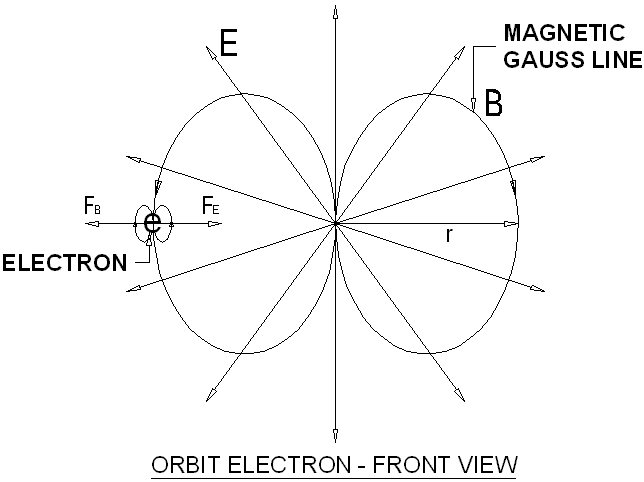Figure ME.2.1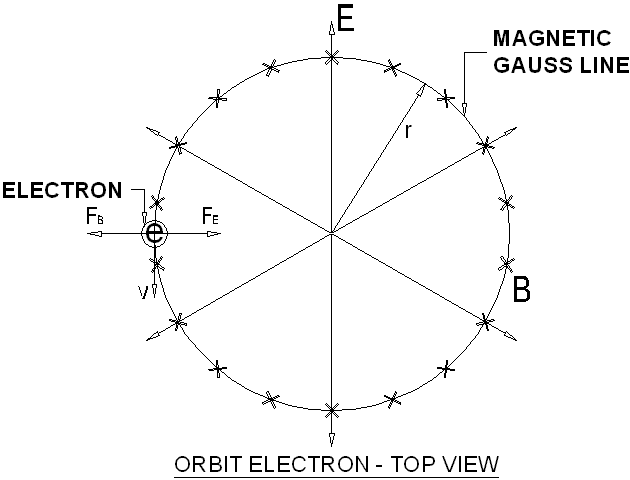Figure ME.2.2   From the figure ME.2.2, the electron travels in a circular path is not due to the centrifugal force. To zoom into a finite view, the electron is actually traveling in the straight line at constant velocity as shown in figure ME.2.3 below. The M&E forces are balance due to the Hall Effect. The circular path is caused by the centralized electric field by the point charge and the toroid magnetic field.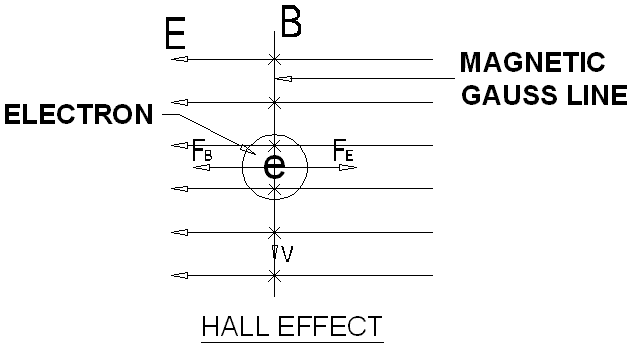Figure ME.2.3   In the Hall effect experiment, the electric field is produced by the flat conducting plat which parallel and straight said from the North to the South. When the M&E forces are balance for the traveling electron, the electron is moving in a straight line from the North to the South according to the direction of the electric field. In the case if the flat conducting plat is rolled into a cylinder and produces cylindrical electric field, under the same circumstance, the electron will move and circulate around the cylinder. This phenomenon explains the same reason for the electron circulates the atom as shown in figure ME.2.2 where the electric field is circular towards the center of the atom.   The atom is made up of nothing but the M&E field. The center of the atom is not stuffed or solid. It does not contain the nucleus, protons or neutrons. The other particles such as electrons, protons and neutrons are also in the form of M&E field, having the similar structure, appear in stable or meta-stable conditions. Unstable particles tend to restructure and deform into the stable photons or particles.   This atom model does not consists of a clear demarcation of the 'nucleus' and the protons or neutrons. It is a lump meta-stable M&E field that provide the volumetric of the atom. Electrons have the size much smaller compare to the atom. The atom attracts and allows the electrons to stay within its M&E field structure. But the electrons never collapse into the 'nucleus' because of  the in-existence of the 'nucleus'.   The atom is noticeable when the transition of electrons interact with the light and touchable due to the differences in density of the M&E field. More descriptions and illustration on the atom 'nucleus' will be discussed in the chapter "Nuclear Physics". ME.3.0        QUANTUM PROPERTIES   ME.3.1        Velocity of Electron   From the Time Dependent Kong Equation, for an electron travels stably in the magnetic gauss line, the (M&E) forces must be balanced. The solution of Time Dependent Kong Equation gives the followings,   Acceleration of Electric force = Acceleration of Magnetic force… eq. ME.3.1   Adding the electron mass, me to the above equation, it becomes,… eq. ME.3.2   Inward pulling force to the center of atom = Outward pushing force due to the traveling effect. The traveling effect is shown by the angular velocity in eq. ME.3.2.   From figure ME.2.2, the equation represents the two forces is, eE = e( v ´ B )                                                                                     … eq. ME.3.3 where   e = charge of electron v = traveling velocity of electron B = magnetic field of magnetic gauss line of atom E = electric field of atom   The velocity is always perpendicular to the magnetic field and the cross product is always equal to the unity. Rearrange eq. ME.3.3, we obtain, vB = E                                                                                               … eq. ME.3.4   The electric field is given as follow,… eq. ME.3.5 where  eo= permittivity constant   Substitute eq. ME.1.12 into eq. ME.3.4 and equate with eq. ME.3.5, we obtain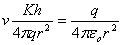… eq. ME.3.6   Solving for the velocity, we obtain,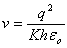… eq. ME.3.7     ME.3.2        Radius of Magnetic Gauss Line   Substitute eq. ME.1.12 into the right side of the eq. ME.3.3, the magnetic force generated is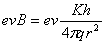.. eq. ME.3.8   From the right side of the eq. ME.3.2, extract the magnetic force portion. The angular traveling force of electron can be also expressed as follow… eq. ME.3.9  where   v = rw   Equating eq. ME.3.8 and eq. ME.3.9 for the magnetic force generated by the traveling effect, we obtain… eq. ME.3.10   Rearrange eq. ME.3.10 to obtain the radius,… eq. ME.3.11   Substitute eq. ME.3.7 into eq. ME.3.11, the radius of the atom is,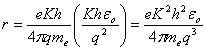… eq. ME.3.12   This is the radius for the magnetic gauss line of the atom. From the solution of the radial equation of the Time Independent Kong Equation, for the discrete magnetic gauss line, the radius is quantized to the n principle orbital number as follow,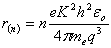, n = 1, 2, 3, …∞                                           … eq. ME.3.13     ME.3.3        Magnetic Gauss Line   The radius for the first magnetic gauss line is developed by letting n = 1. Substitute eq. ME.3.13 into eq. ME.1.12 and expressing the discrete magnetic field in term of the quantum number as follow,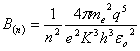… eq. ME.3.14   This is the quantized magnetic field at different radius for different quantum states. The smallest radius has the strongest magnetic field when n = 1. The smallest radius is the last home for the electron, beyond this level, without the support of the magnetic field, the electron is unable to stay in the inner space of the atom. The inner space of the atom is not stuffed, the electrons will never hit on the 'nucleus' and disappear. This is the reason that the electrons do not collapse into the atom but possible to stay at the lowest quantum number.     ME.3.4        Magnetic Dipole Moment of Atom   For an electron travels surrounding the atom, it forms a current loop. The magnetic dipole moment of a charge particle is given as follows, ma = iA                                                                                                … eq. ME.3.15 where m a = magnetic dipole moment of atom= current created by an orbiting electron A = pr2 = the circulation area by the orbiting electron at radius r.   Rearrange eq. ME.3.15, it becomes,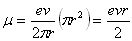… eq. ME.3.16   Substituting eq. ME.3.7 and ME.3.13 into eq. ME.3.16, we obtain, the magnetic dipole moment of the atom at each quantum state is,… eq. ME.3.17      ME.3.5        Orbital Angular Momentum of Atom   The orbital angular momentum is defined as follow, L = mevr                                                                                              … eq. ME.3.18   Substitute eq. ME.3.7 and ME.3.13 into eq. 3.18, the orbital angular momentum of the atom becomes,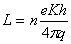… eq. ME.3.19   This orbital angular momentum is only applicable to the planar motion of the electrons as derived in the "Kong Equation". The actual orbital angular momentum corresponds to the atom in considering the tilting effect will be described in detail in the chapter "Quantum of Atom".     ME.3.6        State Energy   From the Kong atom model, the magnetic gauss line is quantized; therefore each quantized state of the magnetic gauss line represents the different state of energy. Figure ME.3.1 shows the diagrammatic representation for the direction of the angular momentum and magnetic moment of atom and electron in an atom model.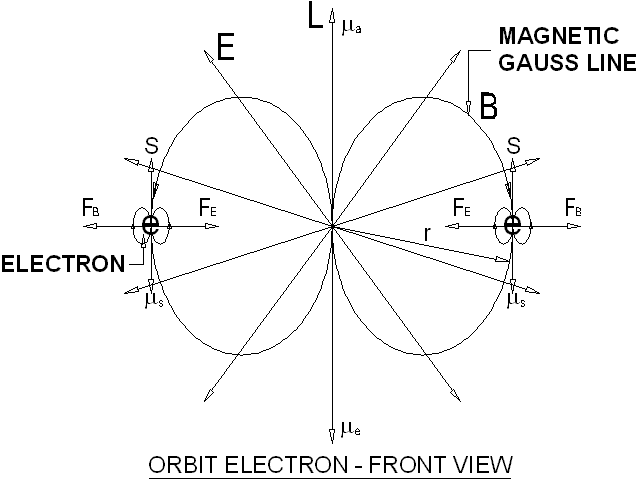Figure ME.3.1   Referring to figure ME.3.1, to keep the electron on track at the correct magnetic dipole orientation; the magnetic potential energy of a magnetic dipole in a magnetic field is given as follow,   U(n) = -ms . B(n)                                                                                  … eq. ME.3.20 where   U(n) = magnetic potential energy of n quantum state ms = spin magnetic dipole moment of electron B(n) = magnetic field of magnetic gauss line at n quantum state   From the chapter "Annihilation and Pair Production", the spin magnetic dipole moment of the electron is given as follow,… eq. ME.3.21   The magnetic potential energy is the dot-product between the magnetic dipole moment of the electron and the magnetic field. The magnetic dipole of the electron is always parallel and same direction to the magnetic field of the atom. The angle of the dot product takes the value zero. Substitute eq. ME.3.21 and eq. ME.3.14 into eq. ME.3.20, we obtain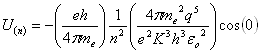… eq. ME.3.22   Rearrange eq. ME.3.22, we obtain,… eq. ME.3.23   The quantum energy of each state of an atom is given by eq. ME.3.23. Eq. ME.3.23 is the magnetic potential energy, which is the energy require to keep the electron on track at the correct orientation.   Eq. ME.3.20 and eq. ME.3.23 also tell that regardless of where the electron comes from, as long as the electron orbits in a quantized magnetic gauss line at the correct orientation, the electron possesses the quantum state energy as it is.   The electron possesses highest state energy when n = 1. Electron does not collapse into the atom ME.4.0        KONG FUNCTION   As explained in the chapter "Kong Frequency and Kong Wavelength", electrons do not travel as wave. Electrons circulate in atom as particle-like. From the magnetic potential energy, eq. ME.3.23 is also the kinetic energy of the orbiting electron. From the special relativity principle, the kinetic energy of a moving electron is expressed as follow,   K = (g - 1)mec2                                                                                    … eq. ME.4.1 where   g = Lorentz factor             c = speed of light   From eq. ME.3.23, drop off the PON, extract the scalar term and equate with eq. ME.4.1, we obtain,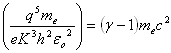… eq. ME.4.2   The charge of atom can be quantized as follow, q = Ze                                                                                                 … eq. ME.4.3 where   Z = Proton number   Substitute eq. ME.4.3 into eq. ME.4.2, we obtain,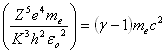… eq. ME.4.4   Rearrange eq. ME.4.4 to obtain the Kong function,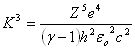... eq. ME.4.5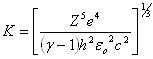… eq. ME.4.6   Eq. ME.4.6 is the Kong function which adhere to the special relativity principle.     ME.4.1        Kong Function At Low Velocity   Substitute eq. ME.4.3 into eq. ME.3.7, the velocity of electron is rearranged as follow to obtain the Kong function,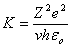… eq. ME.4.7   Substitute eq. ME.4.7 into eq. ME.4.5, we obtain,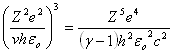Rearrange, we obtain,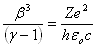… eq. ME.4.8   where   b = v/c = dimensionless speed parameter   Assuming the electron moves at low velocity, expand the Lorentz factor by using the Binomial Theorem and ignore the higher terms of the speed parameter, we have, (g - 1) » ½ b2                                                                                       … eq. ME.4.9   Substitute eq. ME.4.9 into eq.ME.4.8, we obtain the velocity of the orbiting electron at low velocity,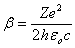… eq. ME.4.10   Substitute eq. ME.4.10 into eq. ME.4.7, we obtain,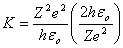K = 2Z                                                                                                … eq. ME.4.11   Eq. ME.4.11 is the Kong function at low velocity. It depends on the proton number of the atom. ME.5.0        HYDROGEN ATOM MODEL   From the sub-chapter ME.3.0, we have developed the quantum properties for the velocity of electron, the quantized radius, the magnetic gauss line, the magnetic dipole moment, the orbital angular momentum and the state energy.   In this chapter, we verify all the equations derived above with the hydrogen atom model. The hydrogen atom has the proton number of Z = 1, the charge of hydrogen atom is, q = e                                                                                                    … eq. ME.5.1   Assuming the electron circulates in the hydrogen atom at low velocity, the Kong function for the hydrogen atom is, K = 2                                                                                                   … eq. ME.5.2     ME.5.1        Electron Traveling Radius   From eq. ME.3.13, the electron travels at radius, r,, n = 1, 2, 3, …∞                                           … eq. ME.3.13   Substituting eq. ME.5.1 and eq. ME.5.2 into eq. ME.3.13, we obtain the radius for the hydrogen atom,, n = 1, 2, 3, …∞                                             … eq. ME.5.3   Eq. ME.5.3 is the quantized electron traveling radius of Hydrogen atom using the Kong atom model. At the ground state n = 1, it reduces to the Bohr radius.     ME.5.2        Kong Magnetic Gauss Line   From eq. ME.3.14, the quantized magnetic field is,… eq. ME.3.14   Substitute eq. ME.5.1 and eq. ME.5.2 into eq. ME.3.14, we obtain the quantized magnetic gauss line,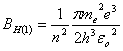… eq. ME.5.4   Replace the quantum state with n = 1, the first quantum magnetic gauss line for the hydrogen atom is given by,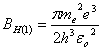BK = 2.35 x 105 Tesla                                                                           … eq. ME.5.5   The quantity BK is named the Kong magnetic gauss line. It is the magnetic field of the hydrogen atom at the ground state.     ME.5.3        Magnetic Dipole Moment   From eq. ME.3.17 the magnetic dipole moment of an atom is given as… eq. ME.3.17    Substitute eq. ME.5.1 and eq. ME.5.2 into eq. ME.3.17, the quantized magnetic dipole moment is,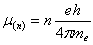… eq. ME.5.6   Replace the quantum state with n = 1, eq. ME.5.6 becomes,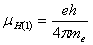… eq. ME.5.7   which is exactly equivalent to the Bohr magneton.     ME.5.4        Orbital Angular Momentum   From eq. ME.3.19, the angular momentum is given as follow,… eq. ME.3.19   Substitute eq. ME.5.1 and eq. ME.5.2 into eq. ME.3.19, the angular momentum for the hydrogen atom becomes,… eq. ME.5.8 where= h / 2p = reduced Plank’s constant   Eq. ME.5.8 is exactly the orbital angular momentum postulated by Niels Bohr.     ME.5.5        State Energy   From eq. ME.3.23, the state energy is given as,… eq. ME.3.23   Substitute eq. ME.5.1 and eq. ME.5.2 into eq. ME.3.23, the state energy for the hydrogen atom is,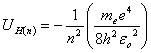… eq. ME.5.9   Eq. ME.5.9 is exactly equivalent to the quantum energy for the hydrogen atom at n state of energy. Replace the quantum state with n = 1, the first quantum energy state for hydrogen atom is,… eq. ME.5.10 ME.6.0        X-RAY SPECTRUM   From eq. ME.3.23, the state energy is given as,… eq. ME.3.23   For electrons transition from higher to lower energy level, photon energy is released. The Photon energy released can be expressed as follow,Rearrange,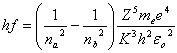… eq. ME.6.1   In the Moseley’s experiment on the characteristic x-ray spectrum, the energy transition for Ka and Kb is used for study. The energy released by electrons when travel from PON n = 2 to n = 1 is categorized as K. Figure ME.6.1 shows the Moseley plot of the Ka line (line 1) and Kb (line 2) respectively of the characteristic x-ray spectra for 21 elements. The electron transition of Ka and Kb are shown in figure ME.6.2.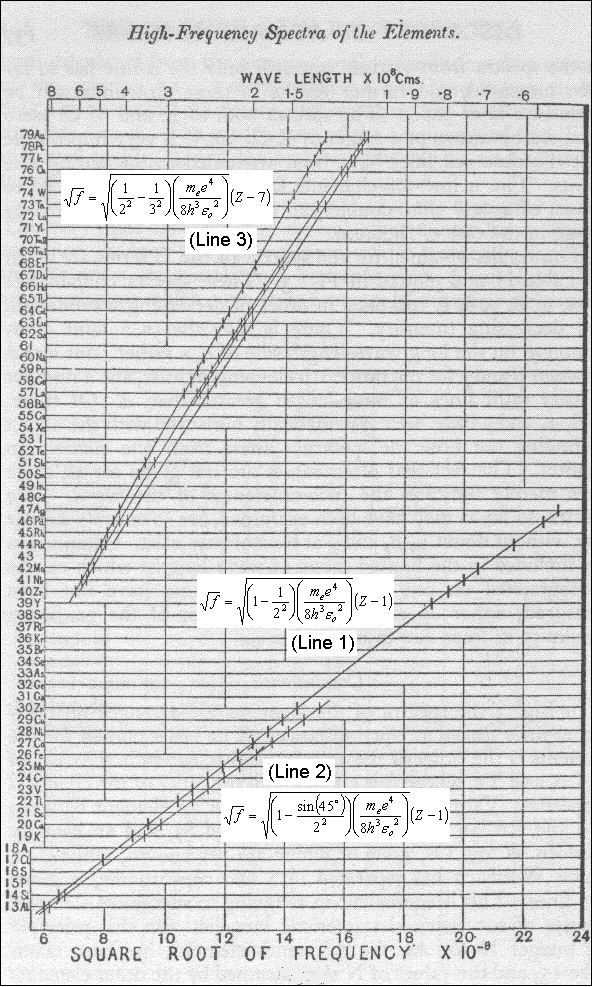Figure ME.6.1Figure ME.6.2     For Ka transition, electrons travels from PON n = 2 to 1. First assume that electrons circulate on PON n = 1 and 2 are travels at low velocity. We can apply eq. ME.4.11 for the Kong function.   Substitute eq. ME.4.11 into eq. ME.6.1, we obtain,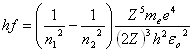Rearrange, we obtain,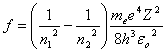… eq. ME.6.2   Eq. ME.6.2 is the basic equation for the Moseley plot. From the chapter "Kong Equation" and "Quantum of Atom", it is developed that the first principle orbital (K-shell) able to house for two numbers of electrons. For the Ka transition, suppose that one of the two electrons in the K-shell is removed, leaving a hole in that shell while another electron still orbiting in the K-shell. The net charge of the atom correspond to the transition electron shall deduct the remaining charge of the orbiting electron. It is appropriate to replace the Z with (Z – 1) because the net charge of the atom is the proton number minus the charge of the orbiting electron at n = 1. And to put n1 = 1 and n2 = 2. Then, eq. ME.6.2 becomes,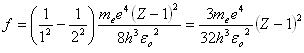… eq. ME.6.3   Square-root both sides of eq. ME.6.3, we obtain,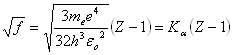… eq. ME.6.4   Simplify eq. ME.6.4, we obtain… eq. ME.6.5 whereEq. ME.6.4 and eq. ME.6.5 are the equations of a straight line. It is in full agreement with the Moseley Plot. The agreement with the Kong atom model is surprisingly good.     ME.6.1        Velocity of Electron   In the above, we first assume that the electrons travel at low velocity. Here, we check on the velocity for conformity. From eq. ME.3.7, the velocity is quoted as follow,… eq. ME.3.7   For an electron circulates at low velocity, substitute eq. ME.4.11 into eq. ME.3.7, we obtain,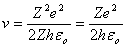… eq. ME.6.6   For the elements at low proton number such as Zinc, where the proton number of Zinc is 30. Replace the Z = 30 in eq. ME.6.6, we obtain,… eq. ME.6.7 where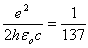The dimensionless speed parameter for electrons circulate in Zinc atom is, b = 30 / 137, 1/2b2 = 0.02398, 1/4b4 = 0.0005748                                                                                … eq. ME.6.8   The ignorance of the higher terms for the expansion of Binomial Theorem for Lorentz factor with small error is still acceptable, where eq. ME.4.9 is still acceptable.     ME.6.2        Higher Proton Number Elements   From the Moseley’s experiment, for elements whose the proton number higher than Molybdenum, such as Silver (Ag), the x-ray spectrum do not fall on the straight line as per eq. ME.6.5. This is due to the value of the Kong function where the velocity of electrons at Ka series is significant high. Eq. ME.4.11 is not appropriate for high proton number elements.   From eq. ME.6.6, we take element silver for example. Silver has the proton number of 47, substitute Z = 47 into eq. ME.6.6, we obtain,… eq. ME.6.9   The dimensionless speed parameter for the electron circulates in Silver atom is, b = 47 / 137, 1/2b2 = 0.05885, 1/4b4 = 0.003463, 1/8b6 = 0.0002038                                                                                … eq. ME.6.10   The accumulated errors for the third and above terms of the expansion of Binomial Theorem for Lorentz factor are significant, where eq. ME.4.9 is not appropriate. Therefore, in the Moseley plot, the high proton number elements do not falls on the straight line of eq. ME.6.5.   From eq. ME.4.5 quoted below,… eq. ME.4.5   Rearrange eq. ME.4.5 to obtain the term of the kinetic energy, we have,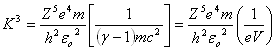… eq. ME.6.11 where   eV = kinetic energy of the circulating electron   From eq. ME.6.11, the Kong function is reverse proportion to the kinetic energy of electrons. We can conclude that when the velocity of electron increases, the kinetic energy of the electron increases. When the kinetic energy of the electron increases, the Kong function decreases.   Then, from eq. ME.6.1 quoted below,… eq. ME.6.1   We can conclude that when the Kong function decreases, the frequency of the released photon increases. Therefore, in the Moseley Plot, the photon frequency increases for the elements of higher proton number. This will be illustrated in figure ME.6.4 later.     ME.6.3        Kb Series   For Kb transition, electrons travels from PON n = 2, SON l = 2 to PON n = 1. Assume that electrons are circulating low velocity. We can apply eq. ME.4.11 for the Kong function. The Kb transition is represented by line 2 in Figure ME.6.1 & ME.6.2.   From the quantum state energy, we derive that… eq. ME.3.23   Substituting q = Ze and only consider the value, we obtain,… eq. ME.6.12   As shown in figure ME.6.2, at PON n = 2, SON l = 1, the quantized energy level of the electron is given by,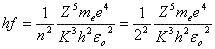... eq. ME.6.13   For an electron travels at PON n = 2, SON l = 2, the tilting angle is 45º to the vertical axis, the energy level is then given by,... eq. ME.6.14   Similar to Ka transition, for the electron at PON n = 2, SON l = 2, falls to PON n = 1, the energy released in represented by Kb transition.   Substituting eq. ME.6.14 into eq. ME.6.2 and replacing n1 = 1, we obtain the following,... eq. ME.6.15   Square-root both sides, we obtain,... eq. ME.6.16   Simplify eq. ME.6.18, we obtain… eq. ME.6.17 whereEq. ME.6.16 and eq. ME.6.17 is the released energy for Kb transition, which is shown by line 2 in figure ME.6.1 and the transition is represented by line 2 in figure ME.6.2. Eq. ME.6.16 is an equations of a straight line. It is in full agreement with the Moseley Plot. The agreement with the Kong atom model is surprisingly good.     ME.6.4        La Series   L-shell is higher that K-shell in the atom. The maximum allowable electrons for L-shell is 6 numbers. Total 8 numbers of electrons for the combination of K-shell and L-shell (KL-shell).   For La transition, electrons travels from PON n = 3 to 2 as shown by line 3 in figure ME.6.2. Assume that electrons circulate on PON n = 3 and 2 are travels at low velocity. We can apply eq. ME.4.11 for the Kong function.   Similar to Ka transition, from eq. ME.6.2 as follow,… eq. ME.6.2   For the La transition, suppose that one of the six electrons in the L-shell is removed, leaving a hole in that shell while the other electrons still orbiting in the L-shell, plus the two electrons circulating in the K-shell, the net charge of the atom correspond to the transition electron shall deduct the remaining charge of the orbiting electron. It is appropriate to replace the Z with (Z – 7) because the net charge of the atom is the proton number minus the charge of the orbiting electron at n = 2 and below. And to put n1 = 2 and n2 = 3. Then, eq. ME.6.2 becomes,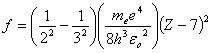… eq. ME.6.18   Square-root both sides of eq. ME.6.18, we obtain,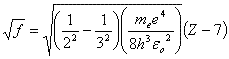… eq. ME.6.19   Simplify eq. ME.6.19, we obtain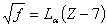… eq. ME.6.20 where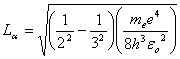Eq. ME.6.19 and eq. ME.6.20 is the released energy for Lα transition, which is shown by line 3 in figure ME.6.1 and the transition is represented by line 3 in figure ME.6.2. Eq. ME.6.19 is an equations of a straight line. It is in full agreement with the Moseley Plot. The agreement with the Kong atom model is surprisingly good.   As described in the chapter "Quantum of Atom", at PON n = 1, the maximum allowable number of electron, Ne is 2. The remaining electrons for PON n = 2 shall be 5 corresponds to the total of 7 electrons. Therefore, the maximum allowable number of electron, Ne shall be 6 for PON n = 2. This is exactly tally with the atom quantum described in the next chapter. ME.7.0        COMPARISON BETWEEN EXPERIMENT AND CALCULATION   From the above, we develop the energy equations for the orbiting electrons in an atom. Here, we compare the experiment data and the calculated value. The experiment collected data and calculated value are tabulated in figure ME.7.1 below. The available data of 39 elements are compared.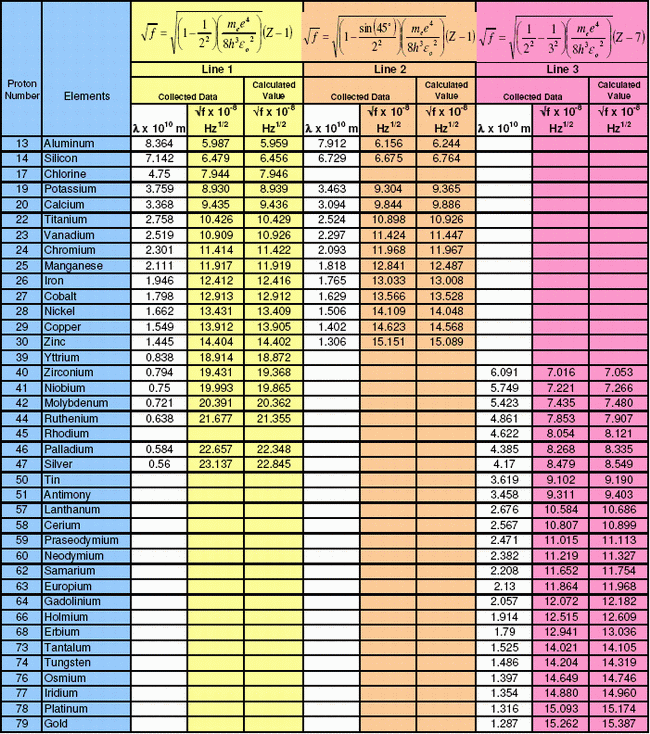Figure ME.7.1   From the data above, a graph is plotted out for easy comparison. The graph is shown in figure ME.7.2 as follow.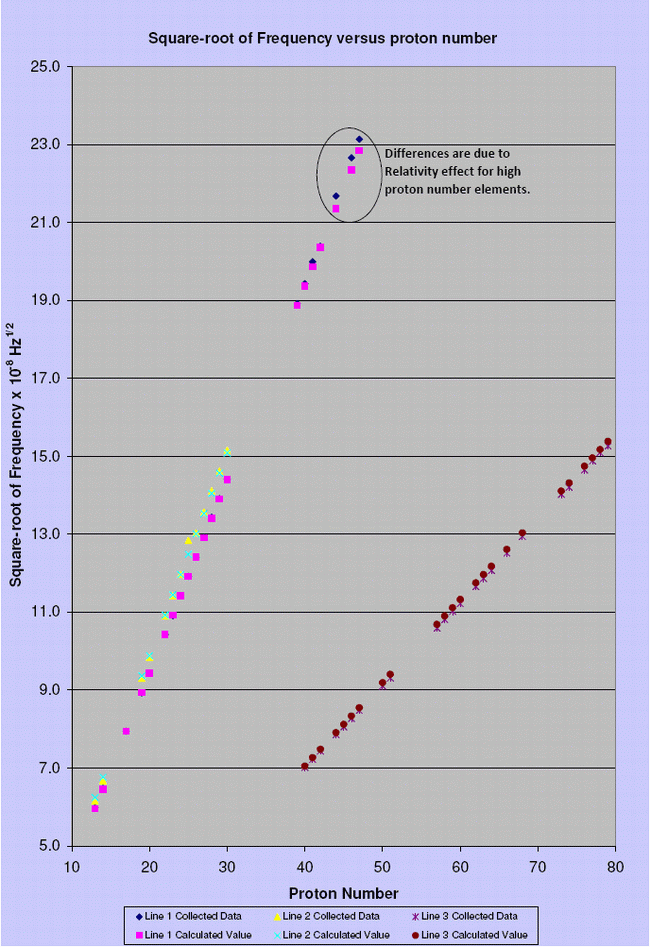Figure ME.7.2   The calculated value is very close to the experiment data. The calculated value is in full agreement with the Moseley Plot. The agreement with the Kong atom model is surprisingly good. DISCUSSIONS AND CONCLUSIONS   The Kong Atom Model gives an alternative understanding of the atom structure. Figure ME.1.1 shows the typical model for all kinds of particles. The atom is made up of nothing but the M&E field. The center of the atom is not stuffed. It does not contain the nucleus, protons or neutrons. The other particles such as electrons, protons and neutrons are also in the form of M&E field, having the similar structure, appear in stable or meta-stable conditions. Unstable particles tend to restructure and deform into the stable photons or particles.   Electrons do not travel as waves in the atom, but it is particle-like and adhering to the special relativity principle. Electrons have the size much smaller compare to the atom. The atom attracts and allows the electrons to stay within its M&E field structure. But the electrons never collapse into the 'nucleus' because of  the in-existence of the 'nucleus'. It can also be described that the atom is the home for electrons.   This atom model does not consists of a clear demarcation of the 'nucleus' and the protons or neutrons. It is a lump meta-stable M&E field that provide the volumetric of the atom. The atom is noticeable when the transition of electrons interact with the light and touchable due to the differences in density of the M&E field.   In developing the Kong atom model, another quantum quantity is introduced, which is the magnetic gauss line. The Kong magnetic gauss line is the magnetic gauss line at the ground state. The magnetic gauss line provides the traveling tracks to the electrons in circulating in the atom.   The quantum mechanics of atom are developed base on the Time Dependent Kong Equation and the Kong atom model. The quantum properties are the velocity of electron, the quantized radius, the magnetic gauss line, the magnetic dipole moment, the orbital angular momentum and the state energy. The Kong function is introduced in the derivations of all the quantum mechanics of atom.   All derivations for the quantum mechanics and the Kong function are verified and tallied with the hydrogen atom model and the Moseley Plot. The Moseley's experiment also provides very good information for the derivations of the atom quantum in the next chapter.   The derivations by using Kong atom model are surprisingly good. The Kong equation and the Kong atom model are acceptable.

This website is originated on 15-Mar-2007,

updated on 4-Jan-2009.